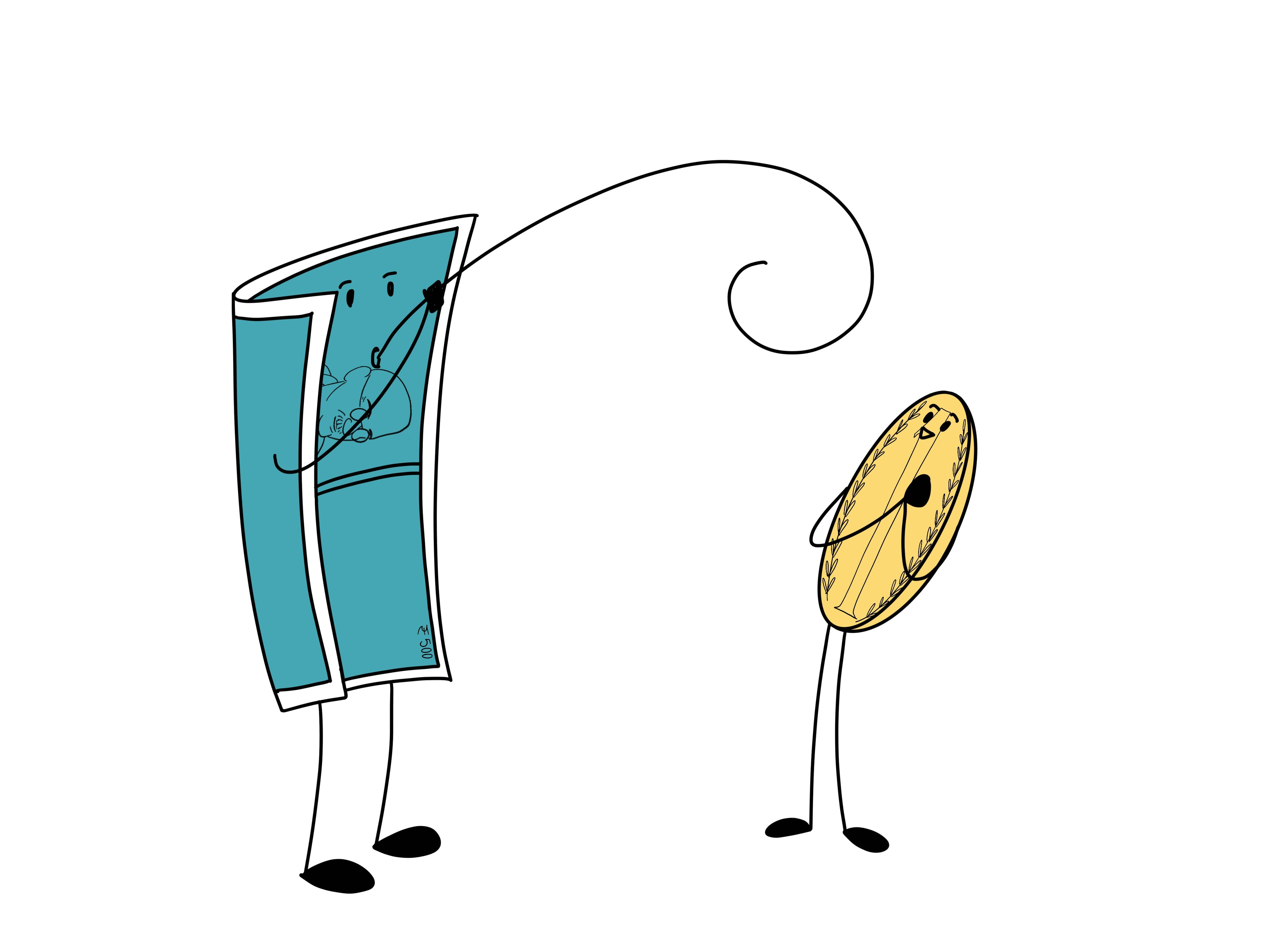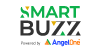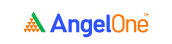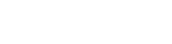# What are Fibonacci Retracement ? How should you use it?

4.8A Fibonacci retracement is a popular tool among technical traders and is based on some key numbers. The origins of the Fibonacci series can be traced back to the ancient Indian mathematical scripts, with some claims dating back to 200 BC. However – in the 12th century - Leonardo Pisano Bigollo, an Italian mathematician who was known to his friends as Fibonacci, discovered Fibonacci numbers.

It is a popular tool that technical traders use to help identify price levels for transactions, stop losses or target prices. These retracement levels also provide support and resistance levels for a stock. However, it really becomes most effective when confirming signals or conditions identified by additional technical analysis tools.

## What is a Fibonacci Retracement?

Fibonacci Retracement is a method of technical analysis for determining support and resistance levels. It is named after the use of the Fibonacci sequence series. It is also based on the idea that markets will retrace a predictable portion of a move, after which they will continue to move in the original direction.

## Construction of Fibonacci Retracement

### Fibonacci Series

Understanding the underlying formula used for construction of Fibonacci Retracement levels helps traders to make prudent decisions, while trading complex scenarios. Fibonacci numbers are a sequence of numbers in which each successive number is the sum of the two previous numbers. The Italian mathematician described a very important correlation between numbers and nature. He introduced this number sequence starting with only two numbers 0 and 1.

1, 1, 2, 3, 5, 8, 13, 21, 34, 55, 89, 144, 233, and so on.

The Fibonacci sequence starts from 0 1 and every number thereafter is built by the sum of the previous two.

Every number in the Fibonacci sequence is 61.8 percent of the next number.

Numbers in the Fibonacci sequence are 38.2 percent of the number after the next in the sequence.

Every number in the Fibonacci sequence is 23.6 percent of the number after the next two numbers in the sequence.

The volume of each part of the Sea Shell matches exactly the Fibonacci numbers sequence. Thus, each part of this shell is 61.8 percent of the next. It works the same way with this aloe flower. If we separate the aloe flower into even particles, following the natural curve of the flower, we will get the same 61.8 percent result. This ratio is not only found in animals and flowers. This ratio is literally everywhere around us. It is in the whirlpool in the sink, in the tornados when looked at through satellite in space or in a water spiral.

The Fibonacci ratio is constantly right in front of us, and we are subliminally used to it. Thus, the human eye considers objects based on the Fibonacci ratio as beautiful and attractive. Also, big corporations like Apple and Toyota have built their logos based on the Fibonacci ratio.

### Strong Uptrend

Defining the primary trend with Fibonacci requires one to measure each pullback of the security. If one sees a series of new highs with retracements of 50 percent or less, the stock is in a strong uptrend.

### Sideways Market

If one sees retracements of 61.8 percent, 78.6 percent or 100 percent, the stock is likely in a basing phase before the next move.

The Fibonacci Retracement is available on most trading platforms, such as Tradingview and MetaTrader. The Fibonacci Retracement Candlesticks are also available on many free online charting sites, such as Investing.com, StockCharts.com and Yahoo! Finance.

### How should you use the Fibonacci retracement levels?

Think of a situation where you wanted to buy a particular stock but you have not been able to do so because of a sharp run up in the stock. In such a situation the most prudent action to take would be to wait for a retracement in the stock. Fibonacci retracement levels such as 61.8%, 38.2%, and 23.6% act as a potential level upto which a stock can correct.

By plotting the Fibonacci retracement levels the trader can identify these retracement levels, and therefore position himself for an opportunity to enter the trade. However please note like any indicator, use the Fibonacci retracement as a confirmation tool.

I would buy a stock only after it has passed the other checklist items. In other words my conviction to buy would be higher if the stock has:

• Formed a recognizable candlestick pattern
• The stop loss coincides with the S&R level
• Volumes are above average

#### Wrapping up

Now that you understand the basics of Fibonacci retracement, let’s learn about Dow theory in the next two chapters.

#### A quick recap

• The Fibonacci series forms the basis for Fibonacci retracement
• A Fibonacci series has many mathematical properties. These mathematical properties are prevalent in many aspects of nature
• Traders believe the Fibonacci series has its application in stock charts as it identified potential retracement levelsTake the quiz for this chapter & mark it complete.

How would you rate this chapter?

Get Information Mindfulness!

Catch-up With Market

News in 60 Seconds.The perfect starter to begin and stay tuned with your learning journey anytime and anywhere.